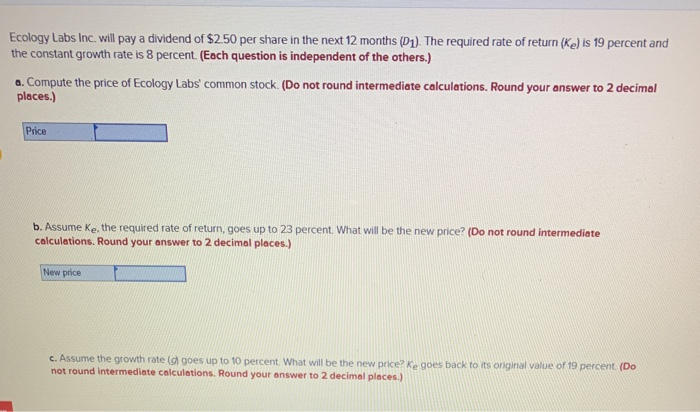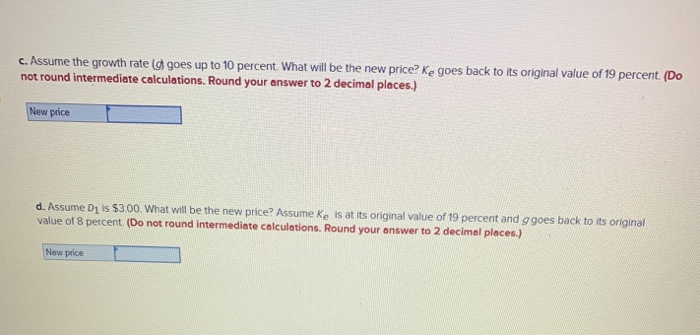### Create an Account

Already have account?

### Forgot Your Password ?

Home / Questions / Ecology Labs Inc. will pay a dividend of \$2.50 per share in the next 12 months (D1). The required...

# Ecology Labs Inc. will pay a dividend of \$2.50 per share in the next 12 months (D1). The required...Ecology Labs Inc. will pay a dividend of \$2.50 per share in the next 12 months (D1). The required rate of return (ke) is 19 percent and the constant growth rate is 8 percent. (Each question is independent of the others.) a. Compute the price of Ecology Labs' common stock. (Do not round intermediate calculations. Round your answer to 2 decimal places.) Price b. Assume ke the required rate of return, goes up to 23 percent. What will be the new price? (Do not round intermediate calculations. Round your answer to 2 decimal places.) New price c. Assume the growth rate a goes up to 10 percent. What will be the new price?Ke goes back to its original value of 19 percent. (Do not round Intermediate calculations. Round your answer to 2 decimal places.)
c. Assume the growth rate goes up to 10 percent. What will be the new price? Ke goes back to its original value of 19 percent. (Do not round intermediate calculations. Round your answer to 2 decimal places.) New price d. Assume D1 is \$3.00 What will be the new price? Assume ke is at its original value of 19 percent and g goes back to its original value of 8 percent (Do not round intermediate calculations. Round your answer to 2 decimal places.) New price

Aug 31 2020 View more View Less

#### Answer (Solved)Subscribe To Get Solution